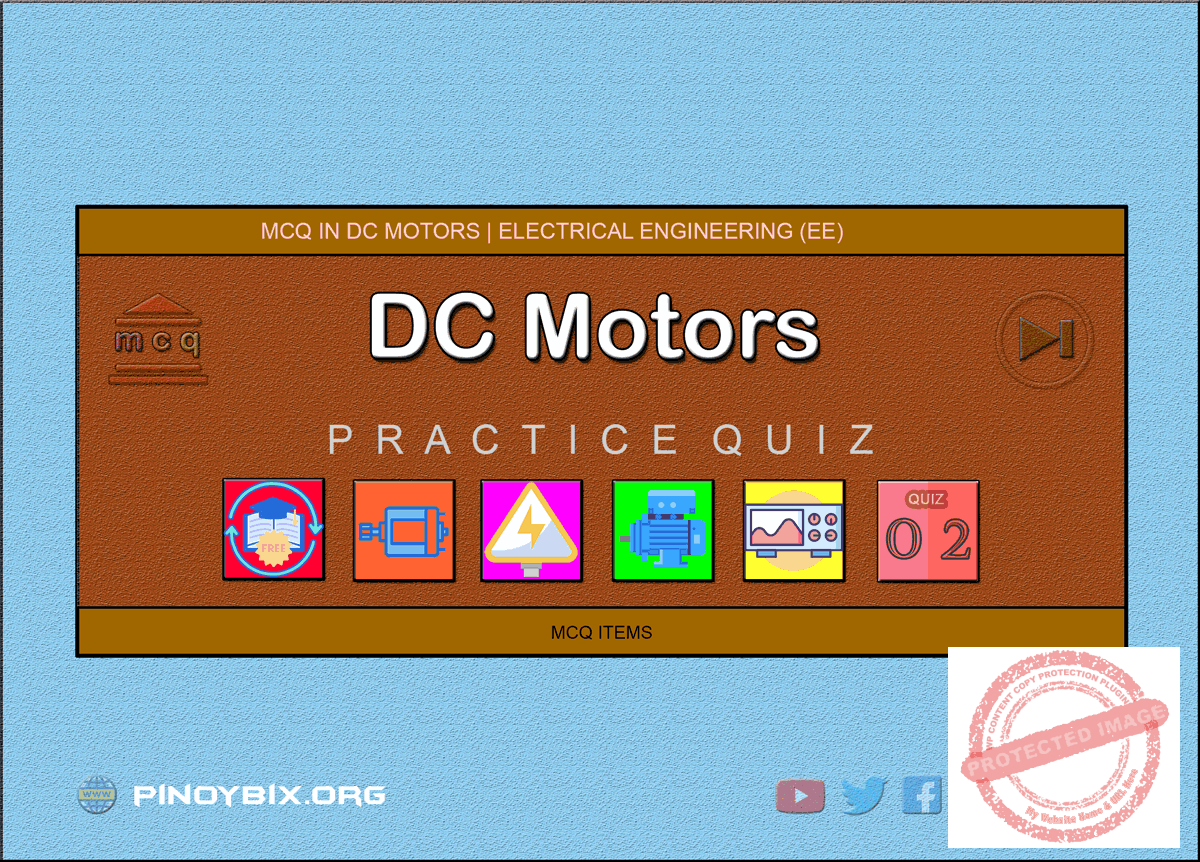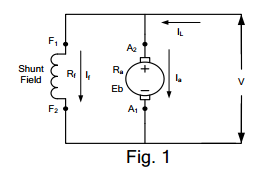# MCQ in DC Motors Part 2 | REE Board Exam

(Last Updated On: July 28, 2020)This is the Multiple Choice Questions Part 2 of the Series in DC Motors as one of the Electrical Engineering topic. In Preparation for the REE Board Exam make sure to expose yourself and familiarize in each and every questions compiled here taken from various sources including but not limited to past Board Exam Questions in Electrical Engineering field, Electrical Engineering Books, Journals and other Electrical Engineering References.

#### Continue Practice Exam Test Questions Part 2 of the Series

Choose the letter of the best answer in each questions.

51.  A d.c. motor develops a torque of 200 N-m at 25 rps. At 20 rps, it will develop a torque of ____ N-m.

A.  200

B.  160

C.  250

D.  128

Solution:

52.  Neglecting saturation, if current taken by a series motor is increased from 10 A to 12 A, the percentage increase in its torque is _____ percent.

A.  20

B.  44

C.  30.5

D.  16.6

Solution:

53.  If load on a d.c. shunt motor is increased, its speed is decreased due primarily to

A.  increase in its flux

B.  decrease in back e.m.f.

C.  increase in armature current

D.  increase in brush drop

Solution:

54.  If the load current and flux of a d.c. motor are held constant and voltage applied across its armature is increased by 10 %, its speed will

B.  remain unchanged

D.  increase by 20%

Solution:

55.  If the pole flux of a d.c. motor approaches zero, its speed will

A.  approach zero

B.  approach infinity

C.  no change due to corresponding change in back e.m.f.

D.  approach a stable value somewhere between zero and infinity

Solution:

56.  If the field current of a loaded shunt motor is suddenly opened

A.  it would race to almost infinite speed

B.  it would draw abnormally high armature current

C.  circuit breaker or fuse will open the circuit before too much damage is done to the motor

D.  torque developed by the motor would be reduced to zero

Solution:

57.  Which of the following d.c. motor would be suitable for drives requiring high starting torque but only fairly constant speed such as crushers?

A.  shunt

B.  series

C.  compound

D.  permanent magnet

Solution:

58.  A d.c. shunt motor is found suitable to drive fans because they require

A.  small torque at start up

B.  large torque at high speeds

C.  practically constant voltage

D.  both A and B

Solution:

59.  Which of the following load would be best driven by a d.c. compound motor?

A.  reciprocating pump

B.  centrifugal pump

C.  electric locomotive

D.  fan

Solution:

60.  As the load is increased, the speed of a d.c. shunt motor

A.  increases proportionately

B.  remains constant

C.  increase slightly

D.  reduces slightly

Solution:

A.  series

B.  shunt

C.  cumulative compound

D.  differential compound

Solution:

62.  The Ta/Ia graph of a d.c. series motor is a

B.  straight line throughout

C.  parabola throughout

Solution:

63.  As compared to shunt and compound motors, series motor has the highest torque because of its comparatively ____ at the start.

A.  lower armature resistance

B.  stronger series field

C.  fewer series turns

D.  larger armature current

Solution:

64.  Unlike shunt motor, it is difficult for a series motor to stall under heavy loading because

A.  it develops high overload torque

B.  its flux remains constant

C.  it slows down considerably

D.  its back e.m.f is reduced to almost zero

Solution:

65.  When load is removed, _____ the motor will run at the highest speed.

A.  shunt

B.  cumulative compound

C.  differential compound

D.  series

Solution:

66.  A series motor is best suited for driving

A.  lathes

B.  cranes and hoists

C.  shears and punches

D.  machine tools

Solution:

67.  A 220 V shunt motor develops a torque of 54 N-m at armature current of 10 A. The torque produced when the armature current is 20 A, is

A.  54 N-m

B.  81 N-m

C.  108 N-m

D.  none of these

Solution:

68.  The d.c. series motor should never be switched on at no load because

A.  the field current is zero

B.  the machine does not pickup

C.  the speed becomes dangerously high

D.  it will take too long to accelerate

Solution:

69.  A shunt d.c. motor works on a.c. mains

A.  unsatisfactory

B.  satisfactorily

C.  none at all

D.  none of these

Solution:

70.  A 200 V, 10 A motor could be rewound for 100 V, 20 A by using ____ as many turns per coil of water having ____ cross-sectional area.

A.  twice, half

B.  thrice, one-third

C.  half, twice

D.  four times, one-fourth

Solution:

71.  The speed of a d.c. motor can be controlled by varying

A.  its flux per pole

B.  resistance of armature circuit

C.  applied voltage

D.  all of these

Solution:

72.  The most efficient method of increasing the speed of a 3.75 kW d.c. shunt motor would be the ____ method.

A.  armature control

B.  flux control

C.  Ward-Leonard

D.  tapped field control

Solution:

73.  Regarding Ward-Leonard system of speed control which statement is false?

A.  it is usually used where wide ad very sensitive speed control is required

B.  it is used for motors having ratings from 750 kW to 4000 kW

C.  capital outlay involved in the system is right since it is uses two extra machine

D.  it has low overall efficiency especially at light loads

Solution:

74.  In the rheostatic method of speed control for a d.c. shunt motor, used of armature diverter makes the method

A.  less wasteful

C.  less expensive

D.  suitable for rapidly changing loads

Solution:

75.  The chief advantage of Ward-Leonard system of d.c. motor speed is that it

A.  can be used for small motors

B.  has high overall efficiency at all speed

C.  gives smooth, sensitive and wide speed control

D.  uses a flywheel to reduce fluctuations in power demand

Solution:

76.  The flux control method using paralleling of field coils when applied to a 4-pole series d.c. motor can give ____ speeds.

A.  2

B.  4

C.  3

D.  6

Solution:

77.  The series-parallel system of speed control of series motor widely used in traction work gives a speed range of about

A.  1:2

B.  1:4

C.  1:3

D.  1:6

Solution:

78.  In practice, regenerative braking is used when

A.  quick motor reversal is desired

C.  controlling elevators, rolling mills and printing presses

D.  other method cannot be used

Solution:

79.  Statement 1. A direct-on-line (DOL) starter is used to start a small d.c. motor because

Statement 2. it limits initial current drawn by the armature circuit.

A.  both statements 1 and 2 are incorrect

B.  both statements 1 and 2 are correct

C.  statement 1 is correct but 2 is wrong

D.  statement 2 is correct but 1 is wrong

Solution:

80.  Ward-Leonard system of speed control is NOT recommended for

A.  wide speed range

B.  constant speed operation

C.  frequent motor reversals

D.  very low speeds

Solution:

81.  Thyristor chopper circuits are employed for

A.  lowering the level of a d.c. voltage

B.  rectifying the a.c. voltage

C.  frequency conversion

D.  providing commutation circuitry

Solution:

82.  An inverter circuit is employed to convert

A.  a.c. voltage into d.c. voltage

B.  d.c. voltage into a.c. voltage

C.  high frequency into law frequency

D.  low frequency into high frequency

Solution:

83.  The phase control rectifiers used for speed of d.c. motors to convert fixed a.c. supply of voltage into

A.  variable d.c. supply voltage

B.  variable a.c. supply voltage

C.  full-rectified a.c voltage

D.  half-rectified a.c. voltage

Solution:

84.  If some of the switching devices in a converter are controlled devices and some are diodes, the converter is called

A.  full converter

B.  semi converter

C.  solid-state copper

D.  d.c. converter

Solution:

85.  A solid-state chopper converts a fixed-voltage d.c. supply into a

A.  variable-voltage a.c. supply

B.  variable-voltage d.c. supply

C.  higher-voltage d.c. supply

D.  lower-voltage a.c. supply

Solution:

86.  The d.c. motor terminal voltage supplied by a solid-state chopper for speed control purposes varies with the duty ratio of the chopper

A.  inversely

B.  linearly

C.  indirectly

D.  parabolically

Solution:

87.  One of the main advantages of Swinburne’s test is that it

A.  is applicable both to shunt and compound motors

B.  needs one running test

C.  is very economical and convenient

D.  ignores any change in iron loss

Solution:

88.  The main disadvantage of Hopkinson’s test for finding efficiency of shunt d.c. motors is that it

B.  ignores any change in loss in iron loss

C.  needs one motor and one generator

D.  requires two identical shunt machines

Solution:

89.  The most economical method of finding noload losses of a large d.c. shunt motor is

A.  Hopkinson’s

B.  Swinburne’s

C.  retardation

D.  Field’s

Solution:

90.  Retardation test on a dc shunt motor is used for finding ____ losses.

A.  stray

B.  copper

C.  friction

D.  iron

Solution:

91.  The main thing common between Hopkinson’s test and field’s test is that both

A.  require two electrically-coupled series motors

B.  need two similar mechanically-coupled motors

C.  use negligible power

D.  are regenerative test

Solution:

92.  The usual test for determining the efficiency of a traction number is the _____  test.

A.  Field’s

B.  retardation

C.  Hopkinson’s

D.  Swinburne’s

Solution:

93.  A dc motor is used to

A.  generate power

B.  change mechanical energy to electrical energy

C.  change electrical energy to mechanical energy

D.  increase energy put into it

Solution:

94.  A dc motor is still used in industrial application because it

A.  is cheap

B.  is simple in construction

C.  provides fine speed control

D.  none of these

Solution:

95.  Carbon brushes are preferable to copper brushes because

A.  they have longer life

B.  they reduce armature reaction

C.  they have lower resistance

D.  they reduce sparking

Solution:

96.  The field poles and armature of a dc machine are laminated to

A.  reduce the weight of the machine

B.  decrease the speed

C.  reduce eddy currents

D.  reduce armature reaction

Solution:

97.  The back emf in a dc motor

A.  opposes the applied voltage

B.  aids the applied voltage

C.  aids the armature current

D.  none of these

Solution:

98.  The value of back emf (Eb) in a dc motor is maximum at

D.  none of these

Solution:

99.  The motor equation is given by

A.  V = Eb – IaRa

B.  V = Eb + IaRa

C.  Eb = IaRa – V

D.  none of these

Solution:

100.  Fig 1 shows the dc shunt motor on no load.The quantity EbIa represents

A.  input power to the armature

B.  copper losses in armature

C.  core losses

D.  total friction and windage and core losses

Solution:

P inoyBIX educates thousands of reviewers and students a day in preparation for their board examinations. Also provides professionals with materials for their lectures and practice exams. Help me go forward with the same spirit.

“Will you subscribe today via YOUTUBE?”

Subscribe

PinoyBIX Engineering. © 2014-2020 All Rights Reserved | How to Donate? |